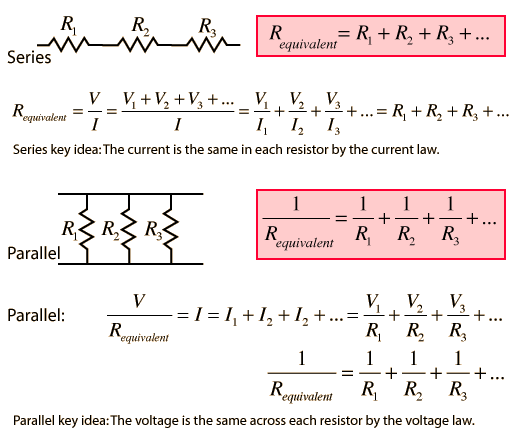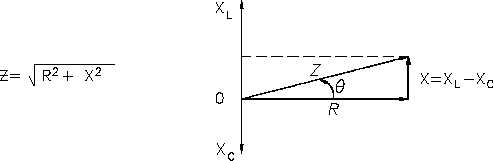1. Unregistered

# Difference between Resistance and Reactance.

difference between resistance and reactance
what is difference between resistance and reactanceReply With Quote

2. kalyani03
Join Date
Aug 2012
Posts
353

## Re: Difference between Resistance and Reactance.Dear friend,

Hope you are doing well.

Here I would like to provide you the difference between the terms RESISTANCE and REACTANCE :-

RESISTANCE;-

->Resistance is defined as the obstruction to the flow of electric charge.

->Resistance is temperature dependent and increases with the increase of temperature.

->Its units are ohm.

->Current is directly proportinal to the drift velocity of free electrons in a conductor and also current is directly protional to the voltage applied across the conductor.This proportionality constant is given by the resistance of the material.->Equivalent resistance of a series combination of resistors is equal to the sum of all the resistance and is greater than the smallest individual resistance.

->Equivalent resistance of a parallel combination of resistors is smaller than the smallest individual resistance.

->If the material through which the current travels is a ohmic material (obeys OHM's law) then the graph between V and I is a straight line and the slope of the straight lie gives the resistance of the material.

->If the material through which the current travels is a non-ohmic material (does not obey OHM's law) then the graph between V and I is not a straight line.

REACTANCE:-

->It is defined as the the opposition or obstruction to a change in voltage due to capacitance or in current due to inductance .

->For example the electric field already setup in that element opposes the change of voltage on that particular element and the magnetic field opposes the change of current.

->Capacitive reactance is the restriction(obstruction) to the change of voltage across an element.

->Inductive reactance is an obstruction to the change of current on an inductive element.

->Ideal resistor-0 reactance

->Ideal inductor-0 resistance

->Ideal capacitor-infinite resistance.

Therefore electrical reactance is different from electrical resistance.

Hope the information helped you.

All the best.Reply With Quote

3. tanveersandhu
Join Date
Jun 2012
Posts
627

## Re: Difference between Resistance and Reactance.

My dear friend.

Ohm's law says that V=IR

Where V is Voltage.

I is Current.

R is Resistance.

We can say that R is inversly proportional to current i.e R=V/I

Hence resistance is opposition to the flow of current.

Every Conductors has some resistance.

Reactance on the other hand is opposition to change in Voltage.Reactance is found in both inductive as well as Capactive circuits.

It is denoted by X.

On the other hand Resistance is denoted by R.

In a circuit in which resistor, capacitor and an Inductor is placed simultaneously there is another term used called as Impedence.Impedence is calculated by adding the Resistance of the circuit with the difference between the Reactance of the circuit.

I hope this information will give you some knowledge about these terms.

Thank You.

Regards
tanveersandhu.Reply With Quote